Successfully reported this slideshow.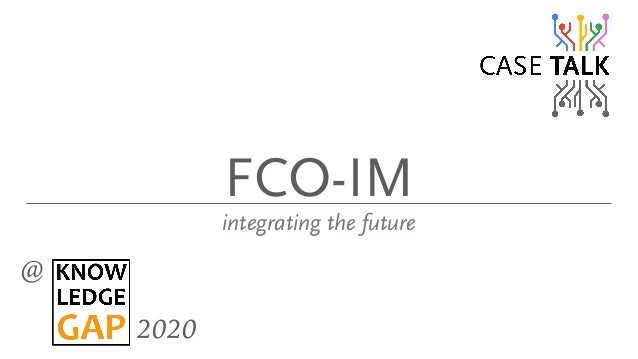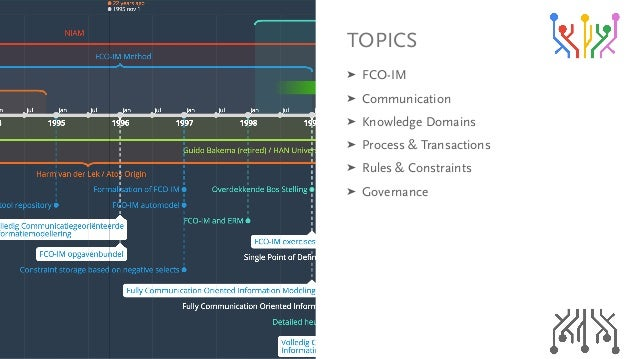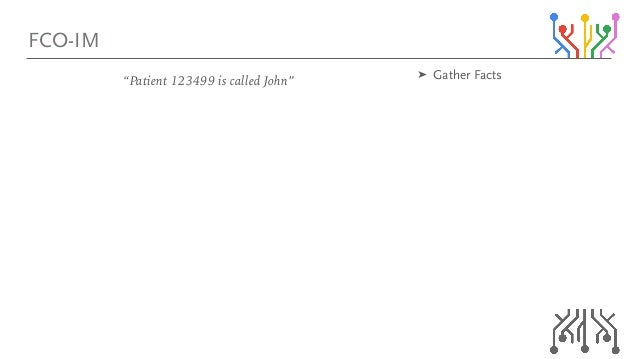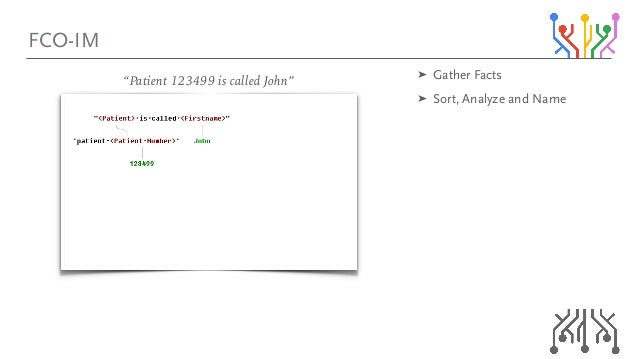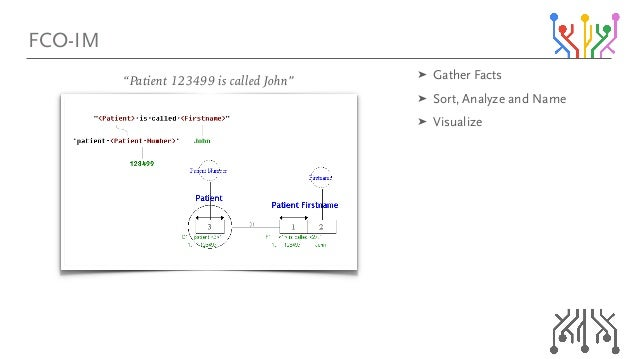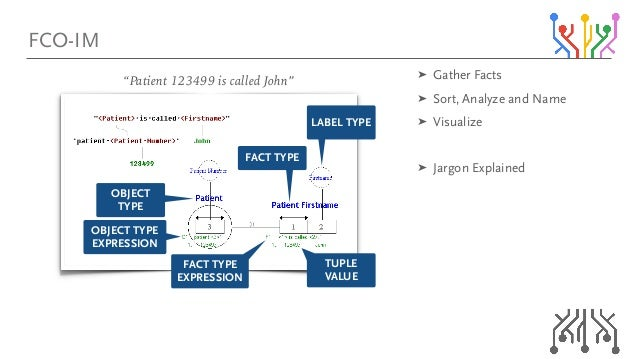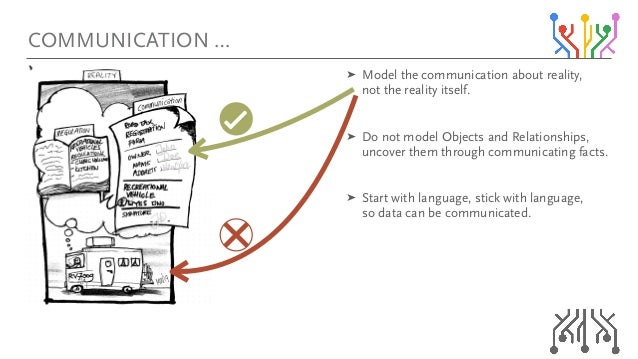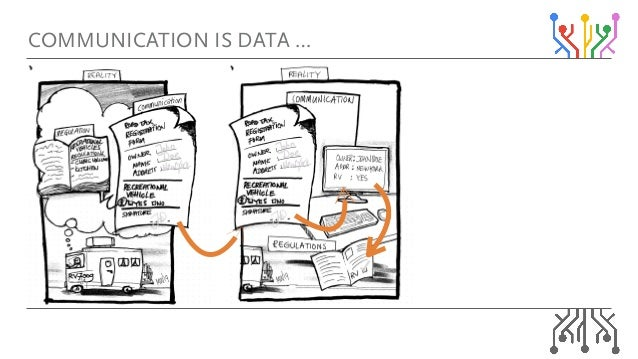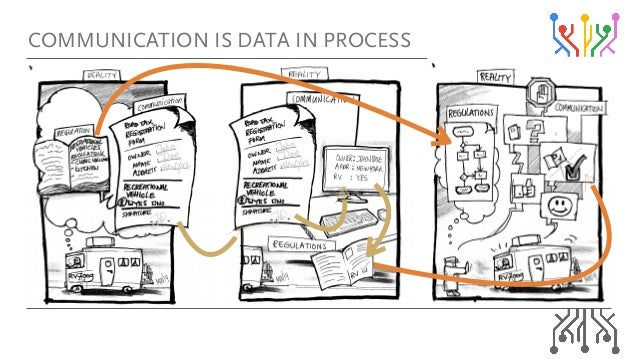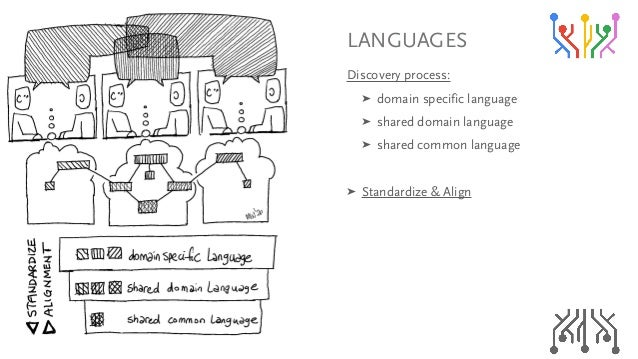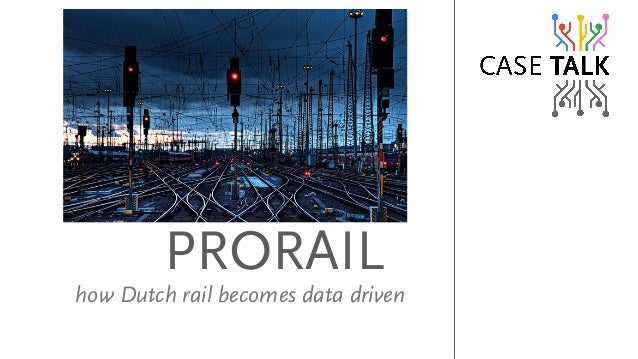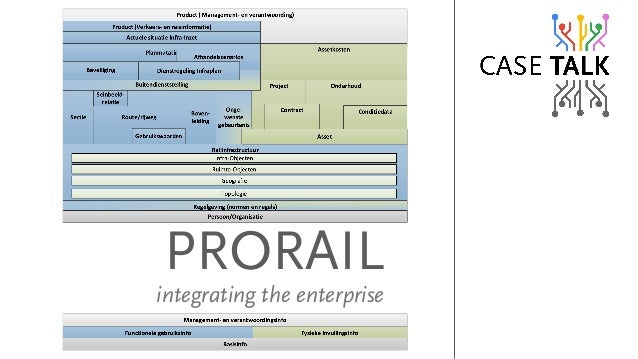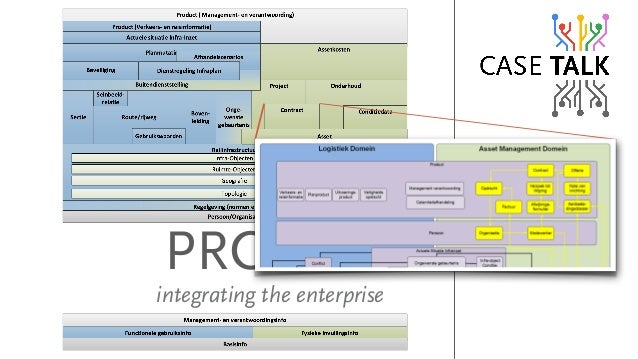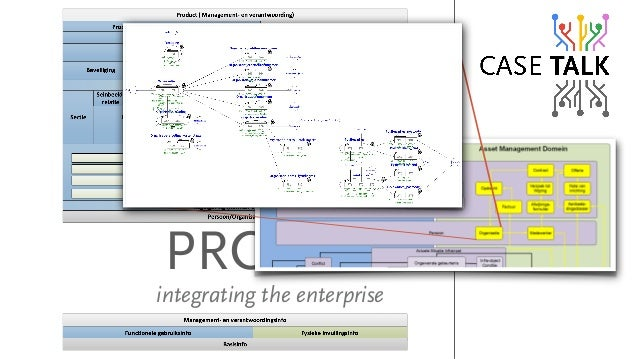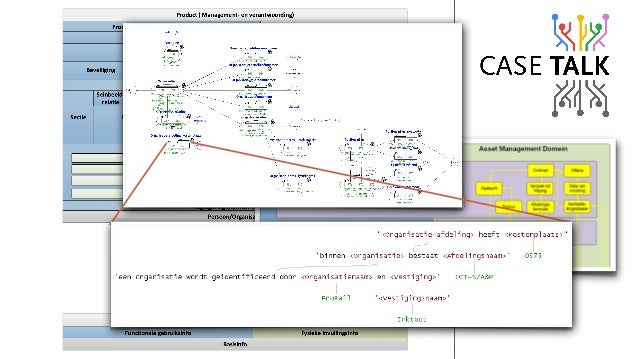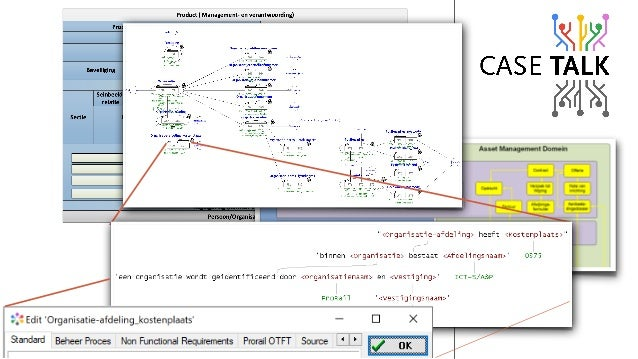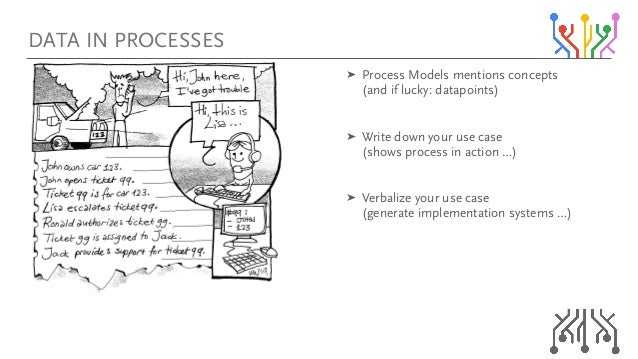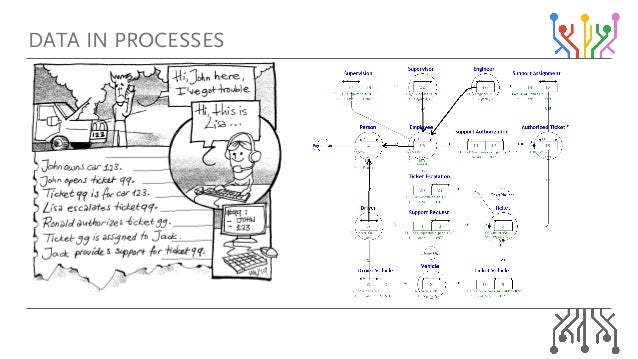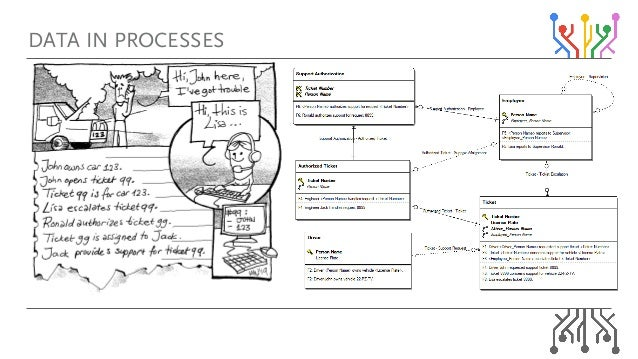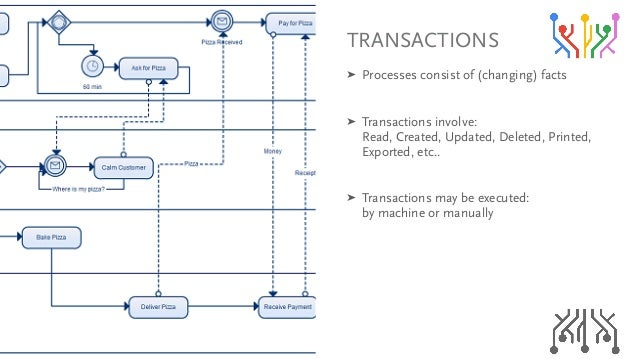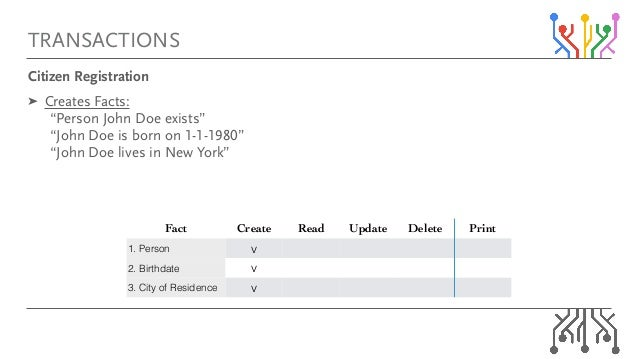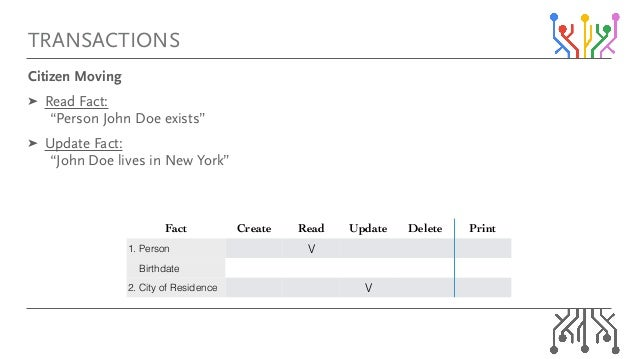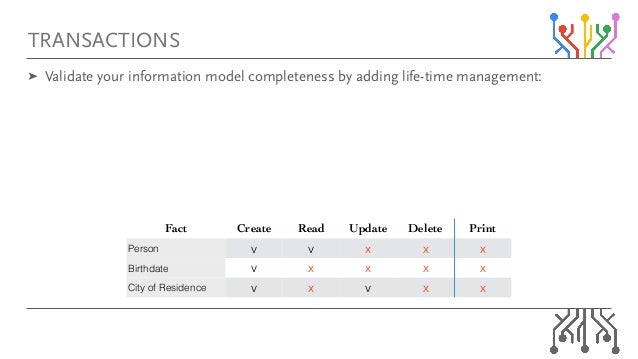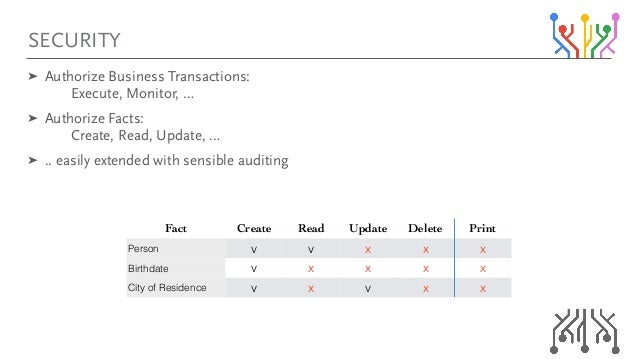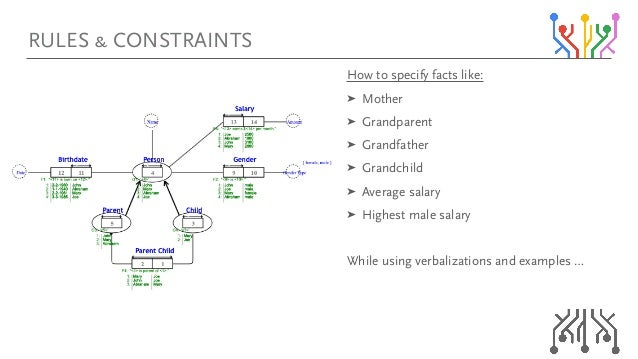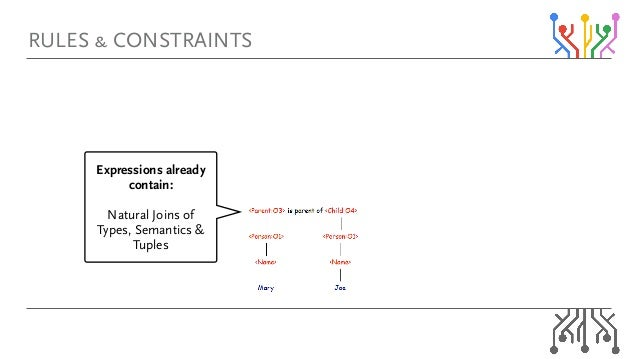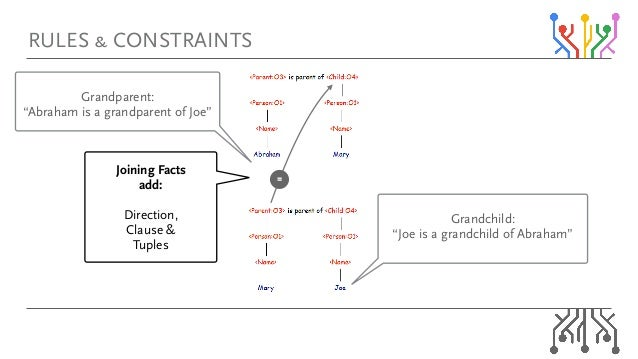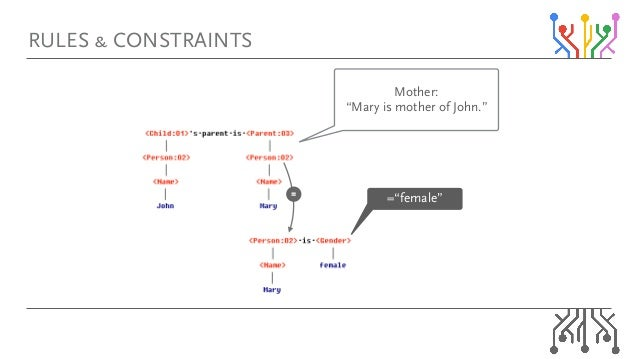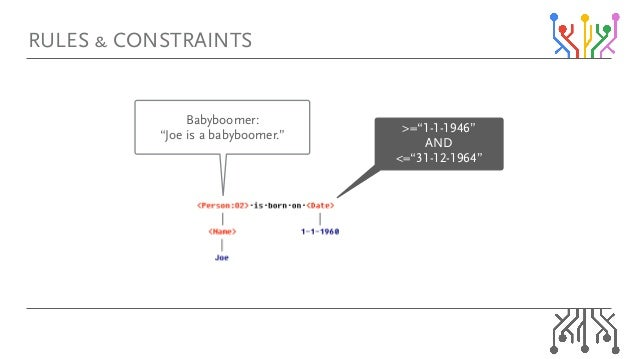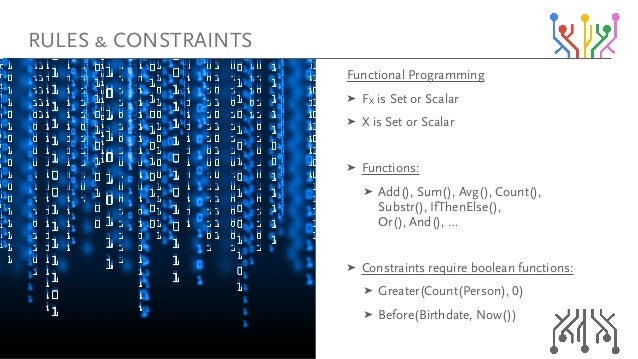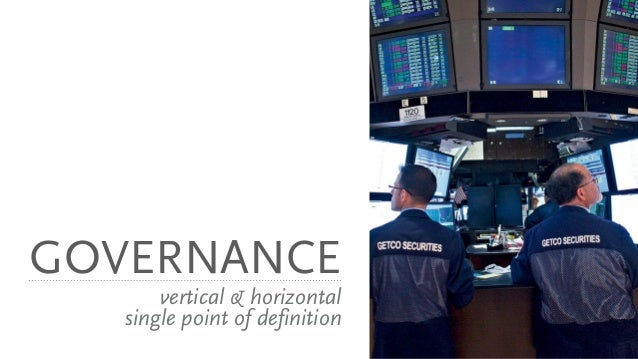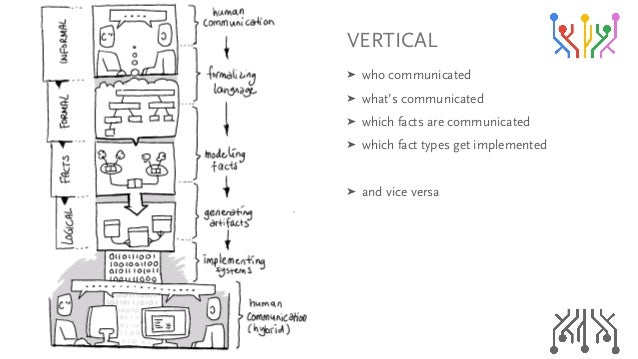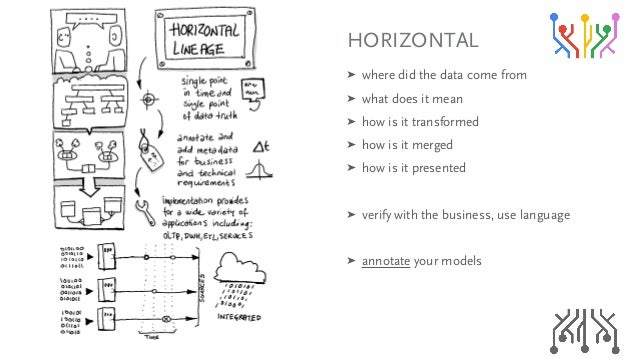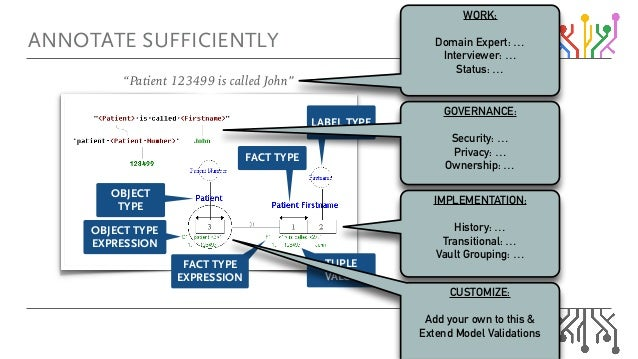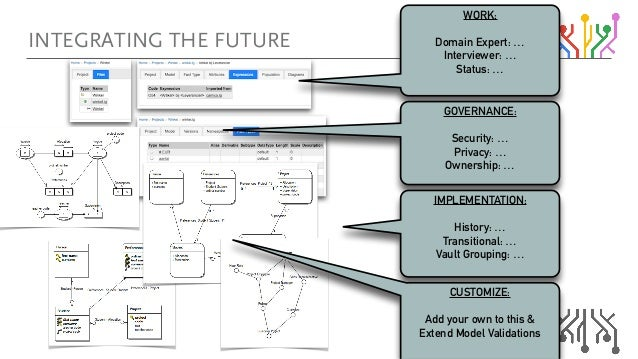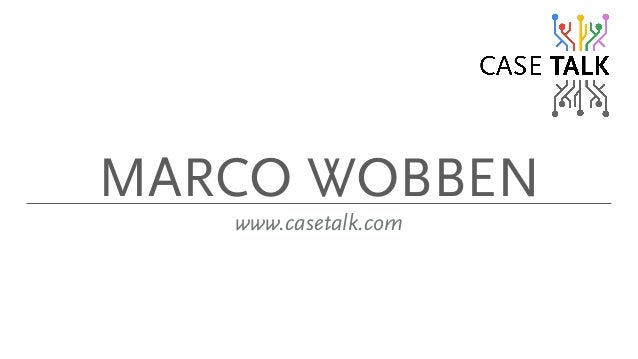Upcoming SlideShare
×

# Fcoim - integrating the future

This presentation was given at the online conference Knowledge Gap 2020. It is about FCO-IM and how it can move forward to integrate Process, Rules & Constraints, and Governance in horizontal and vertical alignment.

• Full Name
Comment goes here.

Are you sure you want to Yes No• Be the first to comment

### Fcoim - integrating the future

1. 1. c`lJfj áåíÉÖê~íáåÖ=íÜÉ=ÑìíìêÉ @ 2020
2. 2. qlmf`p ➤ c`lJfj= ➤ `çããìåáÅ~íáçå= ➤ håçïäÉÇÖÉ=açã~áåë= ➤ mêçÅÉëë=C=qê~åë~Åíáçåë= ➤ oìäÉë=C=`çåëíê~áåíë= ➤ dçîÉêå~åÅÉ
3. 3. c`lJfj “Patient 123499 is called John” ➤ d~íÜÉê=c~Åíë
4. 4. c`lJfj “Patient 123499 is called John” ➤ d~íÜÉê=c~Åíë= ➤ pçêíI=^å~äóòÉ=~åÇ=k~ãÉ
5. 5. c`lJfj “Patient 123499 is called John” ➤ d~íÜÉê=c~Åíë= ➤ pçêíI=^å~äóòÉ=~åÇ=k~ãÉ= ➤ sáëì~äáòÉ
6. 6. c`lJfj i^_bi=qvmb qrmib= s^irb l_gb`q=qvmb= bumobppflk l_gb`q= qvmb c^`q=qvmb c^`q=qvmb= bumobppflk “Patient 123499 is called John” ➤ d~íÜÉê=c~Åíë= ➤ pçêíI=^å~äóòÉ=~åÇ=k~ãÉ= ➤ sáëì~äáòÉ= ➤ g~êÖçå=bñéä~áåÉÇ
7. 7. ➤ jçÇÉä=íÜÉ=ÅçããìåáÅ~íáçå=~Äçìí=êÉ~äáíóI= åçí=íÜÉ=êÉ~äáíó=áíëÉäÑK= ➤ aç=åçí=ãçÇÉä=lÄàÉÅíë=~åÇ=oÉä~íáçåëÜáéëI= ìåÅçîÉê=íÜÉã=íÜêçìÖÜ=ÅçããìåáÅ~íáåÖ=Ñ~ÅíëK= ➤ pí~êí=ïáíÜ=ä~åÖì~ÖÉI=ëíáÅâ=ïáíÜ=ä~åÖì~ÖÉI= ëç=Ç~í~=Å~å=ÄÉ=ÅçããìåáÅ~íÉÇK `ljjrkf`^qflk=Á
8. 8. `ljjrkf`^qflk=fp=a^q^=Á
9. 9. `ljjrkf`^qflk=fp=a^q^=fk=mol`bpp
10. 10. i^kdr^dbp aáëÅçîÉêó=éêçÅÉëëW= ➤ Ççã~áå=ëéÉÅáÑáÅ=ä~åÖì~ÖÉ= ➤ ëÜ~êÉÇ=Ççã~áå=ä~åÖì~ÖÉ= ➤ ëÜ~êÉÇ=Åçããçå=ä~åÖì~ÖÉ= ➤ pí~åÇ~êÇáòÉ=C=^äáÖå
11. 11. Üçï=aìíÅÜ=ê~áä=ÄÉÅçãÉë=Ç~í~=ÇêáîÉå molo^fi
12. 12. molo^fi áåíÉÖê~íáåÖ=íÜÉ=ÉåíÉêéêáëÉ
13. 13. molo^fi áåíÉÖê~íáåÖ=íÜÉ=ÉåíÉêéêáëÉ
14. 14. molo^fi áåíÉÖê~íáåÖ=íÜÉ=ÉåíÉêéêáëÉ
15. 15. molo^fi áåíÉÖê~íáåÖ=íÜÉ=ÉåíÉêéêáëÉ
16. 16. molo^fi áåíÉÖê~íáåÖ=íÜÉ=ÉåíÉêéêáëÉ
17. 17. ➤ mêçÅÉëë=jçÇÉäë=ãÉåíáçåë=ÅçåÅÉéíë= E~åÇ=áÑ=äìÅâóW=Ç~í~éçáåíëF= ➤ têáíÉ=Ççïå=óçìê=ìëÉ=Å~ëÉ= EëÜçïë=éêçÅÉëë=áå=~Åíáçå=ÁF= ➤ sÉêÄ~äáòÉ=óçìê=ìëÉ=Å~ëÉ= EÖÉåÉê~íÉ=áãéäÉãÉåí~íáçå=ëóëíÉãë=ÁF a^q^=fk=mol`bppbp
18. 18. a^q^=fk=mol`bppbp
19. 19. a^q^=fk=mol`bppbp
20. 20. qo^kp^`qflkp ➤ mêçÅÉëëÉë=Åçåëáëí=çÑ=EÅÜ~åÖáåÖF=Ñ~Åíë= ➤ qê~åë~Åíáçåë=áåîçäîÉW= oÉ~ÇI=`êÉ~íÉÇI=réÇ~íÉÇI=aÉäÉíÉÇI=mêáåíÉÇI= bñéçêíÉÇI=ÉíÅKK= ➤ qê~åë~Åíáçåë=ã~ó=ÄÉ=ÉñÉÅìíÉÇW= Äó=ã~ÅÜáåÉ=çê=ã~åì~ääó
21. 21. qo^kp^`qflkp `áíáòÉå=oÉÖáëíê~íáçå= ➤ `êÉ~íÉë=c~ÅíëW= =“mÉêëçå=gçÜå=açÉ=ÉñáëíëÒ= =“gçÜå=açÉ=áë=Äçêå=çå=NJNJNVUMÒ= =“gçÜå=açÉ=äáîÉë=áå=kÉï=vçêâÒ= = = = = Fact Create Read Update Delete Print 1. Person v 2. Birthdate v 3. City of Residence v
22. 22. qo^kp^`qflkp `áíáòÉå=jçîáåÖ= ➤ oÉ~Ç=c~ÅíW= =“mÉêëçå=gçÜå=açÉ=ÉñáëíëÒ= ➤ réÇ~íÉ=c~ÅíW= =“gçÜå=açÉ=äáîÉë=áå=kÉï=vçêâÒ= = = = = = Fact Create Read Update Delete Print 1. Person V Birthdate 2. City of Residence V
23. 23. qo^kp^`qflkp ➤ s~äáÇ~íÉ=óçìê=áåÑçêã~íáçå=ãçÇÉä=ÅçãéäÉíÉåÉëë=Äó=~ÇÇáåÖ=äáÑÉJíáãÉ=ã~å~ÖÉãÉåíW= = = = = = = = = Fact Create Read Update Delete Print Person v v x x x Birthdate v x x x x City of Residence v x v x x
24. 24. pb`rofqv Fact Create Read Update Delete Print Person v v x x x Birthdate v x x x x City of Residence v x v x x ➤ ^ìíÜçêáòÉ=_ìëáåÉëë=qê~åë~ÅíáçåëW= == bñÉÅìíÉI=jçåáíçêI=Á= ➤ ^ìíÜçêáòÉ=c~ÅíëW= == `êÉ~íÉI=oÉ~ÇI=réÇ~íÉI=Á= ➤ KK=É~ëáäó=ÉñíÉåÇÉÇ=ïáíÜ=ëÉåëáÄäÉ=~ìÇáíáåÖ= = = = = =
25. 25. eçï=íç=ëéÉÅáÑó=Ñ~Åíë=äáâÉW= ➤ jçíÜÉê= ➤ dê~åÇé~êÉåí= ➤ dê~åÇÑ~íÜÉê= ➤ dê~åÇÅÜáäÇ= ➤ ^îÉê~ÖÉ=ë~ä~êó= ➤ eáÖÜÉëí=ã~äÉ=ë~ä~êó= tÜáäÉ=ìëáåÖ=îÉêÄ~äáò~íáçåë=~åÇ=Éñ~ãéäÉë=Á oribp=C=`lkpqo^fkqp
26. 26. oribp=C=`lkpqo^fkqp bñéêÉëëáçåë=~äêÉ~Çó= Åçåí~áåW= = k~íìê~ä=gçáåë=çÑ= qóéÉëI=pÉã~åíáÅë=C= qìéäÉë
27. 27. oribp=C=`lkpqo^fkqp = dê~åÇÅÜáäÇW= “gçÉ=áë=~=Öê~åÇÅÜáäÇ=çÑ=^Äê~Ü~ãÒ dê~åÇé~êÉåíW= “^Äê~Ü~ã=áë=~=Öê~åÇé~êÉåí=çÑ=gçÉÒ gçáåáåÖ=c~Åíë= ~ÇÇW= aáêÉÅíáçåI= `ä~ìëÉ=C= qìéäÉë
28. 28. oribp=C=`lkpqo^fkqp = Z“ÑÉã~äÉÒ jçíÜÉêW= “j~êó=áë=ãçíÜÉê=çÑ=gçÜåKÒ
29. 29. oribp=C=`lkpqo^fkqp _~ÄóÄççãÉêW= “gçÉ=áë=~=Ä~ÄóÄççãÉêKÒ [Z“NJNJNVQSÒ= ^ka= YZ“PNJNOJNVSQÒ
30. 30. cìåÅíáçå~ä=mêçÖê~ããáåÖ= ➤ cu=áë=pÉí=çê=pÅ~ä~ê= ➤ u=áë=pÉí=çê=pÅ~ä~ê= ➤ cìåÅíáçåëW= ➤ ^ÇÇEFI=pìãEFI=^îÖEFI=`çìåíEFI= pìÄëíêEFI=fÑqÜÉåbäëÉEFI= lêEFI=^åÇEFI=Á= ➤ `çåëíê~áåíë=êÉèìáêÉ=ÄççäÉ~å=ÑìåÅíáçåëW= ➤ dêÉ~íÉêE`çìåíEmÉêëçåFI=MF= ➤ _ÉÑçêÉE_áêíÜÇ~íÉI=kçïEFF oribp=C=`lkpqo^fkqp
31. 31. dlsbok^k`b îÉêíáÅ~ä=C=Üçêáòçåí~ä= ëáåÖäÉ=éçáåí=çÑ=ÇÉÑáåáíáçå
32. 32. sboqf`^i ➤ ïÜç=ÅçããìåáÅ~íÉÇ= ➤ ïÜ~íÛë=ÅçããìåáÅ~íÉÇ= ➤ ïÜáÅÜ=Ñ~Åíë=~êÉ=ÅçããìåáÅ~íÉÇ= ➤ ïÜáÅÜ=Ñ~Åí=íóéÉë=ÖÉí=áãéäÉãÉåíÉÇ= ➤ ~åÇ=îáÅÉ=îÉêë~
33. 33. elofwlkq^i ➤ ïÜÉêÉ=ÇáÇ=íÜÉ=Ç~í~=ÅçãÉ=Ñêçã= ➤ ïÜ~í=ÇçÉë=áí=ãÉ~å= ➤ Üçï=áë=áí=íê~åëÑçêãÉÇ= ➤ Üçï=áë=áí=ãÉêÖÉÇ= ➤ Üçï=áë=áí=éêÉëÉåíÉÇ= ➤ îÉêáÑó=ïáíÜ=íÜÉ=ÄìëáåÉëëI=ìëÉ=ä~åÖì~ÖÉ= ➤ ~ååçí~íÉ=óçìê=ãçÇÉäë
34. 34. ^kklq^qb=prccf`fbkqiv “Patient 123499 is called John” WORK: Domain Expert: … Interviewer: … Status: … i^_bi=qvmb qrmib= s^irb l_gb`q=qvmb= bumobppflk l_gb`q= qvmb c^`q=qvmb c^`q=qvmb= bumobppflk GOVERNANCE: Security: … Privacy: … Ownership: … IMPLEMENTATION: History: … Transitional: … Vault Grouping: … CUSTOMIZE: Add your own to this & Extend Model Validations
35. 35. fkqbdo^qfkd=qeb=crqrob WORK: Domain Expert: … Interviewer: … Status: … GOVERNANCE: Security: … Privacy: … Ownership: … IMPLEMENTATION: History: … Transitional: … Vault Grouping: … CUSTOMIZE: Add your own to this & Extend Model Validations
36. 36. j^o`l=tl__bk ïïïKÅ~ëÉí~äâKÅçã

### Be the first to comment

•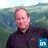#### edwardlangeland

May. 20, 2020

Total views

156

On Slideshare

0

From embeds

0

Number of embeds

99

0

Shares

0